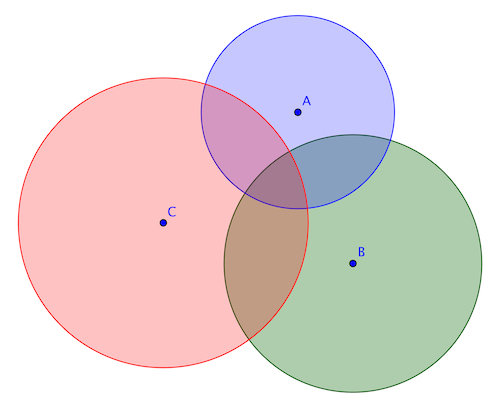# Orthogonal Circles

Geometry Level 4Three mutually orthogonal circles, centered at points A, B, and C, have radii of 6, 8, and 9 respectively. Find the area of $\Delta ABC$.

Two circles are orthogonal to each other if a) they intersect in two points, and b) the two lines tangent to the two circles at each intersection point are perpendicular. So three or more circles are mutually orthogonal if every pair of them are orthogonal to each other.

×

Problem Loading...

Note Loading...

Set Loading...